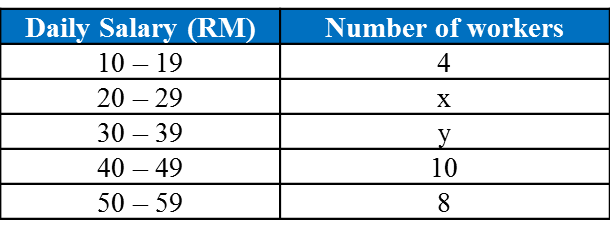# 7.5.3 Statistics, Long Question (Paper 2)

Question 5:
The table shows the cumulative frequency distribution for the distance travelled by 80 children in a competition.(a) Based on the table above, copy and complete the table below.(b) Without drawing an ogive, estimate the interquartile range of this data.

Solution:
(a)(b)
Interquartile range = Third Quartile – First Quartile

Third Quartile class, Q3 = ¾ × 80 = 60
Therefore third quartile class is the class 60 – 69.

First Quartile class, Q1= ¼ × 80 = 20
Therefore first quartile class is the class 30 – 39.

Question 6:
Table shows the daily salary obtained by 40 workers in a construction site.Given that the median daily salary is RM35.5, find the value of x and of y.
Hence, state the modal class.

Solution:Total workers = 40
22 + x + y = 40
x = 18 – y ——(1)

Median daily salary = 35.5
Median class is 30 – 39

$\begin{array}{l}m=L+\left(\frac{\frac{N}{2}-F}{{f}_{m}}\right)c\\ 35.5=29.5+\left(\frac{\frac{40}{2}-\left(4+x\right)}{y}\right)10\\ 6=\left(\frac{16-x}{y}\right)10\\ 6y=160-10x\\ 3y=80-5x----\left(2\right)\end{array}$

Substitute (1) into (2):
3y = 80 – 5(18 – y)
3y = 80 – 90 + 5y
–2y = –10
y = 5
Substitute y = 5 into (1)
x = 18 – 5 = 13
Thus x = 13 and y = 5.

The modal class is 20 – 29 daily salary (RM).

### 1 thought on “7.5.3 Statistics, Long Question (Paper 2)”

1.what class lesson is this?